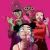views 701

# 12D3

##Gorillaz

I'm 2d
Piano chord
Dictionary

One two D three
One two D three
One two D three
One two D three

Mmm
Mmm
Mmm
Mmm

One two D three
One two D three
One two D three
One two D three

I'm 2d (one two D three)
Won't you buy me (one two D three)
Piano chord (one two D three)
Dictionary (one two D three)

One two D three

I'm 2d (one two D three)
Won't you buy me (one two D three)
Piano chord (one two D three)
Dictionary (one two D three)

One two D three

I'm 2D (one two D three)
Won't you buy me (one two D three)
Piano chord (one two D three)
Dictionary (one two D three)

Add to playlist Size Tab Print Correct

## Comentários

Envie dúvidas, explicações e curiosidades sobre a letra

0 / 500